Home Practice
For learners and parents For teachers and schools
Textbooks
Full catalogue
Pricing SupportLog in

We think you are located in United States. Is this correct?

# 6.5 Area, sine, and cosine rules

## 6.5 Area, sine, and cosine rules (EMBHP)

There are three identities relating to the trigonometric functions that make working with triangles easier:

1. the area rule

2. the sine rule

3. the cosine rule

## The area rule

1. Consider $$\triangle ABC$$:Complete the following:

1. Area $$\triangle ABC$$ = $$\frac{1}{2} \times \ldots \times AC$$

2. $$\sin \hat{B} = \ldots$$ and $$AC = \ldots \times \ldots$$

3. Therefore area $$\triangle ABC$$ = $$\ldots \times \ldots \times \ldots \times \ldots$$

2. Consider $$\triangle A'B'C'$$: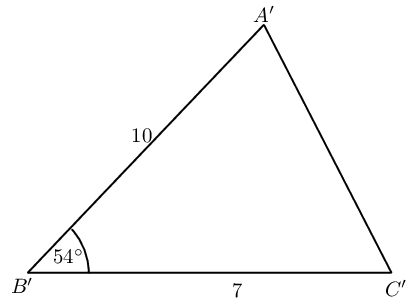Complete the following:

1. How is $$\triangle A'B'C'$$ different from $$\triangle ABC$$?

2. Calculate area $$\triangle A'B'C'$$.

3. Use your results to write a general formula for determining the area of $$\triangle PQR$$: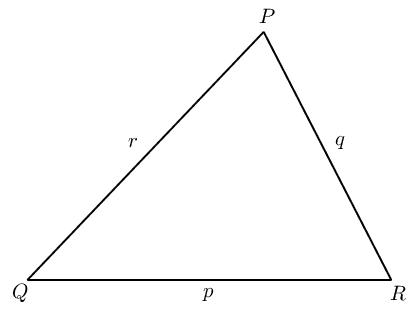For any $$\triangle ABC$$ with $$AB = c, BC = a$$ and $$AC = b$$, we can construct a perpendicular height ($$h$$) from vertex $$A$$ to the line $$BC$$: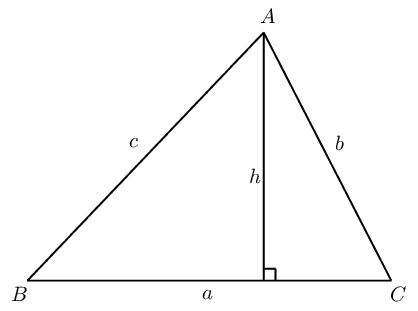In $$\triangle ABC$$:

\begin{align*} \sin \hat{B}&= \frac{h}{c} \\ \therefore h &= c \sin \hat{B} \end{align*}

And we know that

\begin{align*} \text{Area } \triangle ABC &= \frac{1}{2} \times a \times h \\ &= \frac{1}{2} \times a \times c \sin \hat{B} \\ \therefore \text{Area } \triangle ABC &= \frac{1}{2} ac \sin \hat{B} \end{align*}

Alternatively, we could write that

\begin{align*} \sin \hat{C}&= \frac{h}{b} \\ \therefore h &= b \sin \hat{C} \end{align*}

And then we would have that

\begin{align*} \text{Area } \triangle ABC &= \frac{1}{2} \times a \times h \\ &= \frac{1}{2} ab \sin \hat{C} \end{align*}

Similarly, by constructing a perpendicular height from vertex $$B$$ to the line $$AC$$, we can also show that area $$\triangle ABC = \frac{1}{2} bc \sin \hat{A}$$.

The area rule

In any $$\triangle ABC$$:

\begin{align*} \text{Area } \triangle ABC &= \frac{1}{2} bc \sin \hat{A} \\ &= \frac{1}{2} ac \sin \hat{B} \\ &= \frac{1}{2} ab \sin \hat{C} \end{align*}

## Worked example 22: The area rule

Find the area of $$\triangle ABC$$ (correct to two decimal places):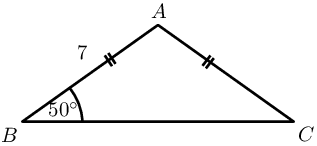### Use the given information to determine unknown angles and sides

$\begin{array}{rll} AB = AC &= 7 & (\text{given}) \\ \therefore \hat{B} = \hat{C} &= \text{50}\text{°} & (\angle \text{s opp. equal sides}) \\ \text{And } \hat{A} &= \text{180}\text{°} - \text{50}\text{°} - \text{50}\text{°} & (\angle \text{s sum of } \triangle ABC) \\ \therefore \hat{A} &= \text{80}\text{°} & \end{array}$

### Use the area rule to calculate the area of $$\triangle ABC$$

Notice that we do not know the length of side $$a$$ and must therefore choose the form of the area rule that does not include this side of the triangle.

In $$\triangle ABC$$:

$\begin{array}{rll} \text{Area } &= \frac{1}{2} bc \sin \hat{A} & \\ &= \frac{1}{2} (7)(7) \sin \text{80}\text{°} & \\ &= \text{24,13} & \end{array}$

Area of $$\triangle ABC = \text{24,13}$$ square units.

## Worked example 23: The area rule

Show that the area of $$\triangle DEF = \frac{1}{2} df \sin \hat{E}$$.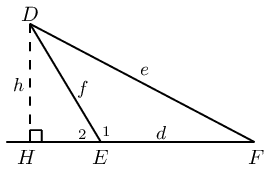### Construct a perpendicular height $$h$$

Draw $$DH$$ such that $$DH \perp EF$$ and let $$DH = h$$, $$D\hat{E}F = \hat{E}_1$$ and $$D\hat{E}H = \hat{E}_2$$.

In $$\triangle DHE$$:

$\begin{array}{rll} \sin \hat{E}_2 &= \dfrac{h}{f} & \\ h &= f \sin (\text{180}\text{°} - \hat{E}_1) & (\angle \text{s on str. line})\\ &= f \sin \hat{E}_1 & \end{array}$

### Use the area rule to calculate the area of $$\triangle DEF$$

In $$\triangle DEF$$:

\begin{align*} \text{Area } &= \frac{1}{2} d \times h \\ &= \frac{1}{2} df \sin \hat{E}_1 \end{align*}

The area rule

In any $$\triangle PQR$$:The area rule states that the area of any triangle is equal to half the product of the lengths of the two sides of the triangle multiplied by the sine of the angle included by the two sides.

## The area rule

Textbook Exercise 6.10

Draw a sketch and calculate the area of $$\triangle PQR$$ given:

$$\hat{Q}=\text{30}\text{°}$$; $$r=10$$ and $$p=7$$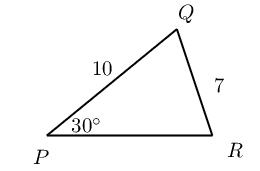$$\hat{R}=\text{110}\text{°}$$; $$p=8$$ and $$q=9$$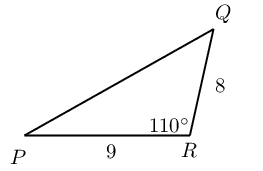Find the area of $$\triangle XYZ$$ given $$XZ = \text{52}\text{ cm}$$, $$XY = \text{29}\text{ cm}$$ and $$\hat{X} = \text{58,9}\text{°}$$.

\begin{align*} \text{Area } \triangle XYZ &= \frac{1}{2} (52)(29) \sin \text{58,9}\text{°} \\ &= \text{645,6}\text{ square units } \end{align*}

Determine the area of a parallelogram in which two adjacent sides are $$\text{10}$$ $$\text{cm}$$ and $$\text{13}$$ $$\text{cm}$$ and the angle between them is $$\text{55}$$$$\text{°}$$.

\begin{align*} \text{Area } &= 2 \left( \frac{1}{2} (13)(10) \sin \text{55}\text{°} \right) \\ &= \text{106,5}\text{ square units } \end{align*}

If the area of $$\triangle ABC$$ is $$\text{5 000}$$ $$\text{m^{2}}$$ with $$a=\text{150}\text{ m}$$ and $$b=\text{70}\text{ m}$$, what are the two possible sizes of $$\hat{C}$$?

\begin{align*} \text{Area } &= \frac{1}{2} (150)(70) \sin \hat{C} \\ \therefore \text{5 000} &= \text{5 250} \sin \hat{C} \\ \therefore \sin \hat{C} &= \frac{\text{5 000} }{\text{5 250} } \\ \therefore \hat{C} &= \text{72,2}\text{°} \\ \text{Or } \hat{C} &= \text{180}\text{°} - \text{72,2}\text{°} \\ &= \text{107,8}\text{°} \end{align*}

### The sine rule (EMBHR)

So far we have only applied the trigonometric ratios to right-angled triangles. We now expand the application of the trigonometric ratios to triangles that do not have a right angle:

## The sine rule

In $$\triangle ABC$$, $$AC = 15$$, $$BC = 11$$ and $$\hat{A} = \text{48}\text{°}$$. Find $$\hat{B}$$.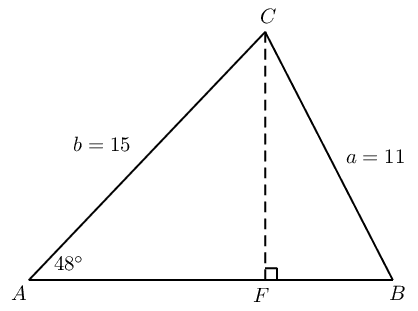1. Method $$\text{1}$$: using the sine ratio

1. Draw a sketch of $$\triangle ABC$$.

2. Construct $$CF \perp AB$$.

3. In $$\triangle CBF$$:

\begin{align*} \frac{CF}{\ldots} &= \sin \hat{B} \\ \therefore CF &= \ldots \times \sin \hat{B} \end{align*}
4. In $$\triangle CAF$$:

\begin{align*} \frac{CF}{15} &= \ldots \\ \therefore CF &= 15 \times \ldots \end{align*}
5. Therefore we have that:

\begin{align*} CF &= 15 \times \ldots \\ \text{and } CF &= \ldots \times \sin \hat{B} \\ \therefore 15 \times \ldots &= \ldots \times \sin \hat{B} \\ \therefore \sin \hat{B} &= \ldots \ldots \ldots \\ \therefore \hat{B} &= \ldots \end{align*}
2. Method $$\text{2}$$: using the area rule

1. In $$\triangle ABC$$:

\begin{align*} \text{Area } \triangle ABC &= \frac{1}{2} AB \times AC \times \ldots \\ &= \frac{1}{2} AB \times \ldots \times \ldots \end{align*}
2. And we also know that

$\text{Area } \triangle ABC = \frac{1}{2} AB \times \ldots \times \sin \hat{B}$
3. We can equate these two equations and solve for $$\hat{B}$$: \begin{align*} \frac{1}{2} AB \times \ldots \times \sin \hat{B} &= \frac{1}{2} AB \times \ldots \times \ldots \\ \therefore \ldots \times \sin \hat{B} &= \ldots \times \ldots \\ \therefore \sin \hat{B} &= \ldots \times \ldots \\ \therefore \hat{B} &= \ldots \end{align*}

3. Use your results to write a general formula for the sine rule given $$\triangle PQR$$: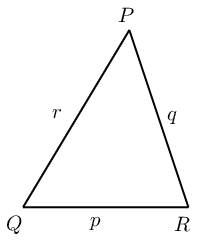For any triangle $$ABC$$ with $$AB = c, BC = a$$ and $$AC = b$$, we can construct a perpendicular height ($$h$$) at $$F$$: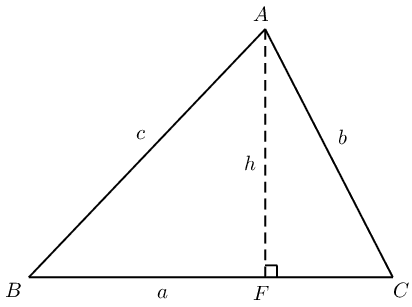Method $$\text{1}$$: using the sine ratio

In $$\triangle ABF$$:

\begin{align*} \sin \hat{B}&= \frac{h}{c} \\ \therefore h &= c \sin \hat{B} \end{align*}

In $$\triangle ACF$$:

\begin{align*} \sin \hat{C}&= \frac{h}{b} \\ \therefore h &= b \sin \hat{C} \end{align*}

We can equate the two equations

\begin{align*} c \sin \hat{B}&= b \sin \hat{C} \\ \therefore \frac{\sin \hat{B}}{b} &= \frac{\sin \hat{C}}{c} \\ \text{or } \frac{b}{\sin \hat{B}} &= \frac{c}{\sin \hat{C}} \end{align*}

Similarly, by constructing a perpendicular height from vertex $$B$$ to the line $$AC$$, we can also show that:

\begin{align*} \frac{\sin \hat{A}}{a} &= \frac{\sin \hat{C}}{c} \\ \text{or } \frac{a}{\sin \hat{A}} &= \frac{c}{\sin \hat{C}} \end{align*}

Similarly, by constructing a perpendicular height from vertex $$B$$ to the line $$AC$$, we can also show that area $$\triangle ABC = \frac{1}{2} bc \sin \hat{A}$$.

Method $$\text{2}$$: using the area rule

In $$\triangle ABC$$:

\begin{align*} \text{Area } \triangle ABC &= \frac{1}{2} ac \sin \hat{B} \\ &= \frac{1}{2} ab \sin \hat{C} \\ \therefore \frac{1}{2} ac \sin \hat{B} &= \frac{1}{2} ab \sin \hat{C} \\ c \sin \hat{B} &= b \sin \hat{C} \\ \frac{\sin \hat{B}}{b} &= \frac{\sin \hat{C}}{c} \\ \text{or } \frac{b}{\sin \hat{B}} &= \frac{c}{\sin \hat{C}} \end{align*}

The sine rule

In any $$\triangle ABC$$:temp text

## Worked example 24: The sine rule

Given $$\triangle TRS$$ with $$S\hat{T}R = \text{55}\text{°}$$, $$TR = 30$$ and $$R\hat{S}T = \text{40}\text{°}$$, determine $$RS, ST$$ and $$T\hat{R}S$$.

### Draw a sketch

Let $$RS = t$$, $$ST = r$$ and $$TR = s$$.### Find $$T\hat{R}S$$ using angles in a triangle

$\begin{array}{rll} T\hat{R}S + R\hat{S}T + S\hat{T}R &= \text{180}\text{°} & (\angle \text{s sum of } \triangle TRS) \\ \therefore T\hat{R}S &= \text{180}\text{°} - \text{40}\text{°} - \text{55}\text{°} & \\ &= \text{85}\text{°} & \end{array}$

### Determine $$t$$ and $$r$$ using the sine rule

\begin{align*} \frac{t}{\sin \hat{T}} &= \frac{s}{\sin \hat{S}} \\ \frac{t}{\sin \text{55}\text{°}} &= \frac{30}{\sin \text{40}\text{°}} \\ \therefore t &= \frac{30}{\sin \text{40}\text{°}} \times \sin \text{55}\text{°} \\ &= \text{38,2} \end{align*}\begin{align*} \frac{r}{\sin \hat{R}} &= \frac{s}{\sin \hat{S}} \\ \frac{r}{\sin \text{85}\text{°}} &= \frac{30}{\sin \text{40}\text{°}} \\ \therefore r &= \frac{30}{\sin \text{40}\text{°}} \times \sin \text{85}\text{°} \\ &= \text{46,5} \end{align*}

## Worked example 25: The sine rule

Prove the sine rule for $$\triangle MNP$$ with $$MS \perp NP$$.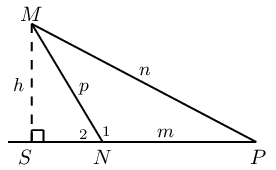### Use the sine ratio to express the angles in the triangle in terms of the length of the sides

In $$\triangle MSN$$:

$\begin{array}{rll} \sin \hat{N}_2&= \frac{h}{p} & \\ \therefore h &= p \sin \hat{N}_2 & \\ \text{and } \hat{N}_2 &= \text{180}\text{°}- \hat{N}_1 & \angle \text{s on str. line} \\ \therefore h &= p \sin (\text{180}\text{°}- \hat{N}_1) & \\ &= p \sin \hat{N}_1 \end{array}$

In $$\triangle MSP$$:

\begin{align*} \sin \hat{P}&= \frac{h}{n} \\ \therefore h &= n \sin \hat{P} \end{align*}

### Equate the two equations to derive the sine rule

\begin{align*} p \sin \hat{N}_1 &= n \sin \hat{P} \\ \therefore \frac{\sin \hat{N}_1}{n} &= \frac{\sin \hat{P}}{p} \\ \text{or } \frac{n}{\sin \hat{N}_1} &= \frac{p}{\sin \hat{P}} \end{align*}

The ambiguous case

If two sides and an interior angle of a triangle are given, and the side opposite the given angle is the shorter of the two sides, then we can draw two different triangles ($$\triangle NMP$$ and $$\triangle NMP'$$), both having the given dimensions. We call this the ambiguous case because there are two ways of interpreting the given information and it is not certain which is the required solution.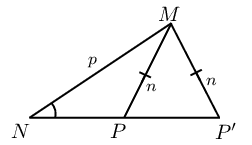## Worked example 26: The ambiguous case

In $$\triangle ABC$$, $$AB = 82$$, $$BC = 65$$ and $$\hat{A} = \text{50}\text{°}$$. Draw $$\triangle ABC$$ and find $$\hat{C}$$ (correct to one decimal place).

### Draw a sketch and identify the ambiguous case

We notice that for the given dimensions of $$\triangle ABC$$, the side $$BC$$ opposite $$\hat{A}$$ is shorter than $$AB$$. This means that we can draw two different triangles with the given dimensions.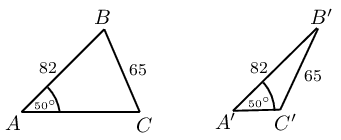### Solve for unknown angle using the sine rule

In $$\triangle ABC$$:

\begin{align*} \frac{\sin \hat{A}}{BC} &= \frac{\sin \hat{C}}{AB} \\ \frac{\sin \text{50}\text{°}}{65} &= \frac{\sin \hat{C}}{82} \\ \therefore \frac{\sin \text{50}\text{°}}{65} \times 82 &= \sin \hat{C} \\ \therefore \hat{C} &= \text{75,1}\text{°} \end{align*}

In $$\triangle A'B'C'$$:

We know that $$\sin (\text{180} - \hat{C}) = \sin \hat{C}$$, which means we can also have the solution

\begin{align*} \hat{C'}&= \text{180}\text{°} - \text{75,1}\text{°} \\ &= \text{104,9}\text{°} \end{align*}

Both solutions are correct.

## Worked example 27: Lighthouses

There is a coastline with two lighthouses, one on either side of a beach. The two lighthouses are $$\text{0,67}$$ $$\text{km}$$ apart and one is exactly due east of the other. The lighthouses tell how close a boat is by taking bearings to the boat (a bearing is an angle measured clockwise from north). These bearings are shown on the diagram below.

Calculate how far the boat is from each lighthouse.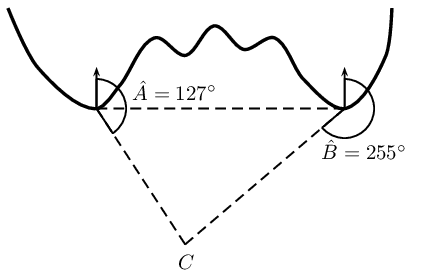We see that the two lighthouses and the boat form a triangle. Since we know the distance between the lighthouses and we have two angles we can use trigonometry to find the remaining two sides of the triangle, the distance of the boat from the two lighthouses.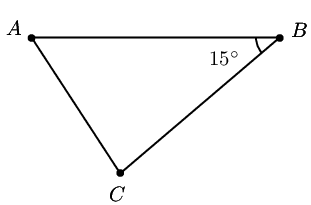We need to determine the lengths of the two sides $$AC$$ and $$BC$$. We can use the sine rule to find the missing lengths.

\begin{align*} \frac{BC}{\sin\hat{A}} &= \frac{AB}{\sin\hat{C}} \\ BC &= \frac{AB \cdot \sin\hat{A}}{\sin\hat{C}} \\ &= \frac{(\text{0,67}\text{ km})\sin \text{37}\text{°}}{\sin \text{128}\text{°}} \\ &= \text{0,51}\text{ km} \end{align*}\begin{align*} \frac{AC}{\sin\hat{B}} &= \frac{AB}{\sin\hat{C}} \\ AC &= \frac{AB \cdot \sin\hat{B}}{\sin\hat{C}} \\ &= \frac{(\text{0,67}\text{ km})\sin \text{15}\text{°}}{\sin \text{128}\text{°}} \\ &= \text{0,22}\text{ km} \end{align*}

## Sine rule

Textbook Exercise 6.11

Find all the unknown sides and angles of the following triangles:

$$\triangle PQR$$ in which $$\hat{Q}=\text{64}\text{°}$$; $$\hat{R}=\text{24}\text{°}$$ and $$r=3$$

\begin{align*} \frac{q}{\sin \hat{Q}} &= \frac{r}{\sin \hat{R}} \\ \frac{q}{\sin \text{64}\text{°} } &= \frac{3}{\sin\text{24}\text{°}} \\ q &= \frac{3\sin \text{64}\text{°} }{\sin\text{24}\text{°}} \\ &= \text{6,6}\text{ units } \\ \hat{P} &= \text{180}\text{°} - \text{64}\text{°} - \text{24}\text{°} \\ &= \text{92}\text{°} \\ \frac{p}{\sin \hat{P}} &= \frac{r}{\sin \hat{R}} \\ \frac{p}{\sin \text{92}\text{°} } &= \frac{3}{\sin\text{24}\text{°}} \\ p &= \frac{3\sin \text{92}\text{°} }{\sin\text{24}\text{°}} \\ &= \text{7,4}\text{ units } \end{align*}

$$\triangle KLM$$ in which $$\hat{K}=\text{43}\text{°}$$; $$\hat{M}=\text{50}\text{°}$$ and $$m=1$$

\begin{align*} \frac{m}{\sin \hat{M}} &= \frac{l}{\sin \hat{L}} \\ \frac{1}{\sin \text{50}\text{°} } &= \frac{l}{\sin\text{87}\text{°}} \\ l &= \frac{\sin \text{87}\text{°} }{\sin\text{50}\text{°}} \\ &= \text{1,3}\text{ units } \\ \hat{L} &= \text{180}\text{°} - \text{50}\text{°} - \text{43}\text{°} \\ &= \text{87}\text{°} \\ \frac{k}{\sin \hat{K}} &= \frac{m}{\sin \hat{M}} \\ \frac{k}{\sin \text{43}\text{°} } &= \frac{1}{\sin\text{50}\text{°}} \\ k &= \frac{\sin \text{43}\text{°} }{\sin\text{50}\text{°}} \\ &= \text{0,89}\text{ units } \end{align*}

$$\triangle ABC$$ in which $$\hat{A} = \text{32,7}\text{°}$$; $$\hat{C} = \text{70,5}\text{°}$$ and $$a = \text{52,3}$$

\begin{align*} \frac{c}{\sin \hat{C}} &= \frac{a}{\sin \hat{A}} \\ \frac{c}{\sin \text{70,5}\text{°} } &= \frac{\text{52,3}}{\sin\text{32,7}\text{°}} \\ c &= \frac{\text{52,3} \sin \text{70,5}\text{°} }{\sin\text{32,7}\text{°}} \\ &= \text{91,3}\text{ units } \\ \hat{B} &= \text{180}\text{°} - \text{70,5}\text{°} - \text{32,7}\text{°} \\ &= \text{76,8}\text{°} \\ \frac{b}{\sin \hat{B}} &= \frac{a}{\sin \hat{A}} \\ \frac{b}{\sin \text{76,8}\text{°} } &= \frac{\text{52,3}}{\sin\text{32,7}\text{°}} \\ b &= \frac{\text{52,3} \sin \text{76,8}\text{°} }{\sin\text{32,7}\text{°}} \\ &= \text{94,3}\text{ units } \end{align*}

$$\triangle XYZ$$ in which $$\hat{X}=\text{56}\text{°}$$; $$\hat{Z}=\text{40}\text{°}$$ and $$x=50$$

\begin{align*} \frac{x}{\sin \hat{X}} &= \frac{z}{\sin \hat{Z}} \\ \frac{50}{\sin \text{56}\text{°} } &= \frac{z}{\sin\text{40}\text{°}} \\ z &= \frac{\text{50} \sin \text{40}\text{°} }{\sin\text{56}\text{°}} \\ &= \text{38,8}\text{ units } \\ \hat{Y} &= \text{180}\text{°} - \text{56}\text{°} - \text{40}\text{°} \\ &= \text{84}\text{°} \\ \frac{y}{\sin \hat{Y}} &= \frac{x}{\sin \hat{X}} \\ \frac{y}{\sin \text{84}\text{°} } &= \frac{\text{50}}{\sin\text{40}\text{°}} \\ y &= \frac{\text{50} \sin \text{84}\text{°} }{\sin\text{56}\text{°}} \\ &= \text{60}\text{ units } \end{align*}

In $$\triangle ABC$$, $$\hat{A}=\text{116}\text{°}$$; $$\hat{C}=\text{32}\text{°}$$ and $$AC = \text{23}\text{ m}$$. Find the lengths of the sides $$AB$$ and $$BC$$.

\begin{align*} \hat{B} &= \text{180}\text{°} - \text{116}\text{°} - \text{32}\text{°} \\ &= \text{32}\text{°} \\ \frac{a}{\sin \hat{A}} &= \frac{b}{\sin \hat{B}} \\ \frac{a}{\sin \text{116}\text{°} } &= \frac{23}{\sin\text{32}\text{°}} \\ a &= \frac{\text{23} \sin \text{116}\text{°} }{\sin\text{32}\text{°}} \\ &= \text{39,01}\text{ units } \\ b &= \text{23}\text{ units } \end{align*}

In $$\triangle RST$$, $$\hat{R}=\text{19}\text{°}$$; $$\hat{S}=\text{30}\text{°}$$ and $$RT = \text{120}\text{ km}$$. Find the length of the side $$ST$$.

\begin{align*} \frac{s}{\sin \hat{S}} &= \frac{r}{\sin \hat{R}} \\ \frac{120}{\sin \text{30}\text{°} } &= \frac{ST}{\sin\text{19}\text{°}} \\ ST &= \frac{\text{120} \sin \text{19}\text{°} }{\sin\text{30}\text{°}} \\ &= \text{78,1}\text{ km } \end{align*}

In $$\triangle KMS$$, $$\hat{K}=\text{20}\text{°}$$; $$\hat{M}=\text{100}\text{°}$$ and $$s = \text{23}\text{ cm}$$. Find the length of the side $$m$$.

\begin{align*} \hat{S} &= \text{180}\text{°} - \text{100}\text{°} - \text{20}\text{°} \\ &= \text{60}\text{°} \\ \frac{m}{\sin \hat{M}} &= \frac{s}{\sin \hat{S}} \\ \frac{m}{\sin \text{100}\text{°} } &= \frac{23}{\sin \text{60}\text{°}} \\ m &= \frac{\text{23} \sin \text{100}\text{°} }{\sin\text{60}\text{°}} \\ &= \text{26,2}\text{ units } \end{align*}

In $$\triangle ABD$$, $$\hat{B} = \text{90}\text{°}$$, $$AB = \text{10}\text{ cm}$$ and $$A\hat{D}B = \text{40}\text{°}$$. In $$\triangle BCD$$, $$\hat{C} = \text{106}\text{°}$$ and $$C\hat{D}B = \text{15}\text{°}$$. Determine $$BC$$.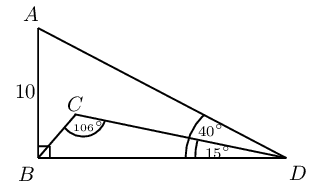\begin{align*} \frac{BD}{\sin \text{50}\text{°} } &= \frac{10}{\sin\text{40}\text{°}} \\ BD &= \frac{\text{10} \sin \text{50}\text{°} }{\sin\text{40}\text{°}} \\ &= \text{11,92}\text{ units } \\ \frac{BC}{\sin \text{15}\text{°} } &= \frac{\text{11,92}}{\sin \text{106}\text{°}} \\ BC &= \frac{\text{11,92} \sin \text{15}\text{°} }{\sin\text{106}\text{°}} \\ &= \text{3,2}\text{ units } \end{align*}

In $$\triangle ABC$$, $$\hat{A} = \text{33}\text{°}$$, $$AC = \text{21}\text{ mm}$$ and $$AB = \text{17}\text{ mm}$$. Can you determine $$BC$$?

No, not enough information is given

## The cosine rule

If a triangle is given with two sides and the included angle known, then we can not solve for the remaining unknown sides and angles using the sine rule. We therefore investigate the cosine rule:

In $$\triangle ABC, AB = 21, AC = 17$$ and $$\hat{A} = \text{33}\text{°}$$. Find $$\hat{B}$$.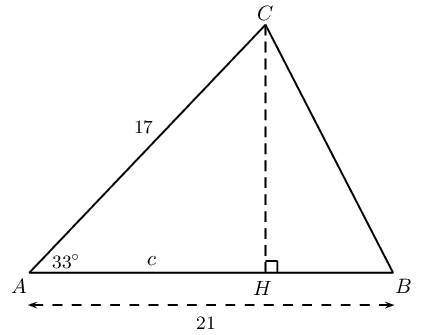1. Determine $$CB$$:

1. Construct $$CH \perp AB$$.

2. Let $$AH = c$$ and therefore $$HB = \ldots$$

3. Applying the theorem of Pythagoras in the right-angled triangles:

In$$\triangle CHB$$:

\begin{align*} CB^2 &= BH^2 + CH^2 \\ &= (\ldots)^2 + CH^2 \\ &= 21^2 -(2)(21)c + c^2 + CH^2 \ldots \ldots (1) \end{align*}

In $$\triangle CHA$$:

\begin{align*} CA^2 &= c^2 + CH^2 \\ 17^2 &= c^2 + CH^2 \ldots \ldots (2) \end{align*}

Substitute equation $$(2)$$ into equation $$(1)$$:

$CB^2 = 21^2 -(2)(21)c + 17^2$

Now $$c$$ is the only remaining unknown. In $$\triangle CHA$$:

\begin{align*} \frac{c}{17} &= \cos \text{33}\text{°} \\ \therefore c &= 17 \cos \text{33}\text{°} \end{align*}

Therefore we have that

\begin{align*} CB^2 &= 21^2 -(2)(21)c + 17^2 \\ &= 21^2 -(2)(21)(17 \cos \text{33}\text{°}) + 17^2 \\ &= 21^2 + 17^2 -(2)(21)(17) \cos \text{33}\text{°} \\ &= \text{131,189}\ldots \\ \therefore CB &= \text{11,5} \end{align*}
2. Use your results to write a general formula for the cosine rule given $$\triangle PQR$$: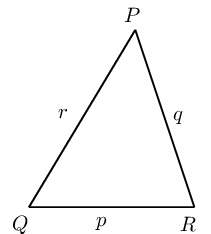The cosine rule relates the length of a side of a triangle to the angle opposite it and the lengths of the other two sides.

Consider $$\triangle ABC$$ with $$CD \perp AB$$: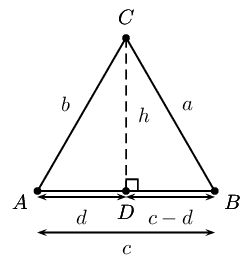In $$\triangle DCB$$: $$a^2 = (c-d)^2 + h^2$$ from the theorem of Pythagoras.

In $$\triangle ACD$$: $$b^2 = d^2 + h^2$$ from the theorem of Pythagoras.

Since $$h^2$$ is common to both equations we can write: \begin{align*} a^2 &= (c-d)^2 + h^2 \\ \therefore h^2 &= a^2 - (c-d)^2 \\ \text{And } b^2 &= d^2 + h^2 \\ \therefore h^2 &= b^2 - d^2 \\ \therefore b^2 - d^2 &= a^2 - (c-d)^2 \\ a^2 &= b^2 + (c^2 - 2cd + d^2) - d^2 \\ &= b^2 + c^2 - 2cd \end{align*}

In order to eliminate $$d$$ we look at $$\triangle ACD$$, where we have: $$\cos\hat{A} = \frac{d}{b}$$. So, $$d = b \cos \hat{A}$$.

Substituting back we get: $${a}^{2}={b}^{2}+{c}^{2}-2bc \cos \hat{A}$$.

The cosine rule

In any $$\triangle ABC$$: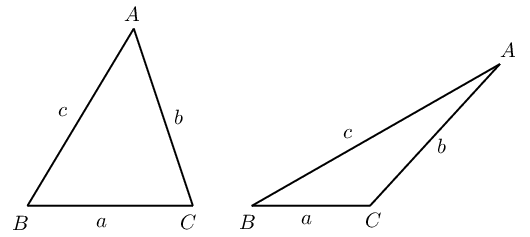temp text

## Worked example 28: The cosine rule

Determine the length of $$QR$$.### Use the cosine rule to solve for the unknown side

\begin{align*} QR^2 &= PR^2 + QP^2 -2 (PR)(QP) \cos \hat{P} \\ &= 4^2 + 13^2 -2 (4)(13) \cos \text{70}\text{°} \\ &= \text{149,42}\ldots \\ \therefore QR &= \text{12,2} \end{align*}

$$QR = \text{12,2}\text{ cm}$$

## Worked example 29: The cosine rule

Determine $$\hat{A}$$.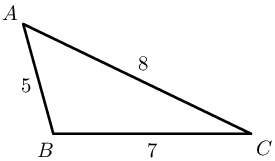Applying the cosine rule:

\begin{align*} {a}^{2} &= {b}^{2}+{c}^{2}-2bc \cos \hat{A} \\ \therefore \cos\hat{A} &= \frac{{b}^{2}+{c}^{2}-{a}^{2}}{2bc} \\ &= \frac{{8}^{2}+{5}^{2}-{7}^{2}}{2 \cdot 8 \cdot 5} \\ &= \text{0,5} \\ \therefore \hat{A} &= \text{60}\text{°} \end{align*}

It is very important:

• not to round off before the final answer as this will affect accuracy;
• to take the square root;
• to remember to give units where applicable.

How to determine which rule to use:

1. Area rule:

• if no perpendicular height is given
2. Sine rule:

• if no right angle is given
• if two sides and an angle are given (not the included angle)
• if two angles and a side are given
3. Cosine rule:

• if no right angle is given
• if two sides and the included angle are given
• if three sides are given

## The cosine rule

Textbook Exercise 6.12

Solve the following triangles (that is, find all unknown sides and angles):

$$\triangle ABC$$ in which $$\hat{A}=\text{70}\text{°}$$; $$b=4$$ and $$c=9$$

\begin{align*} {a}^{2} &= {b}^{2}+{c}^{2}-2bc \cos \hat{A} \\ {a}^{2} &= {4}^{2}+{9}^{2}-2(9)(4) \cos \hat{\text{70}\text{°}} \\ &= \text{72,374} \ldots \\ \therefore a &= \text{8,5} \end{align*} \begin{align*} {c}^{2} &= {b}^{2}+{a}^{2}-2ba \cos \hat{C} \\ {9}^{2} &= {4}^{2}+{\text{8,5}}^{2}-2(4)(\text{8,5}) \cos \hat{C} \\ \therefore \hat{C} &= \text{83,9}\text{°} \end{align*} \begin{align*} \hat{B} &= \text{180}\text{°} - \text{70}\text{°} -\text{83,9}\text{°} \\ \therefore \hat{B} &= \text{26,1}\text{°} \end{align*}

$$\triangle RST$$ in which $$RS=14$$; $$ST=26$$ and $$RT=16$$

\begin{align*} {r}^{2} &= {s}^{2}+{t}^{2}-2st \cos \hat{R} \\ {26}^{2} &= {16}^{2}+{\text{14}}^{2}-2(16)(\text{14}) \cos \hat{R} \\ \therefore \hat{R} &= \text{120}\text{°} \end{align*} \begin{align*} {s}^{2} &= {r}^{2}+{t}^{2}-2rt \cos \hat{S} \\ {16}^{2} &= {26}^{2}+{\text{14}}^{2}-2(26)(\text{14}) \cos \hat{S} \\ \therefore \hat{S} &= \text{32,2}\text{°} \end{align*} \begin{align*} \hat{T} &= \text{180}\text{°} - \text{120}\text{°} -\text{32,2}\text{°} \\ \therefore \hat{T} &= \text{27,8}\text{°} \end{align*}

$$\triangle KLM$$ in which $$KL=5$$; $$LM=10$$ and $$KM=7$$

\begin{align*} {m}^{2} &= {k}^{2}+{l}^{2}-2kl \cos \hat{M} \\ {5}^{2} &= {10}^{2}+{\text{7}}^{2}-2(10)(\text{7}) \cos \hat{M} \\ \therefore \hat{M} &= \text{27,7}\text{°} \end{align*} \begin{align*} {l}^{2} &= {k}^{2}+{m}^{2}-2km \cos \hat{L} \\ {7}^{2} &= {10}^{2}+{\text{5}}^{2}-2(10)(\text{5}) \cos \hat{L} \\ \therefore \hat{L} &= \text{40,5}\text{°} \end{align*} \begin{align*} \hat{K} &= \text{180}\text{°} - \text{27,7}\text{°} -\text{40,5}\text{°} \\ \therefore \hat{K} &= \text{111,8}\text{°} \end{align*}

$$\triangle JHK$$ in which $$\hat{H}=\text{130}\text{°}$$; $$JH=13$$ and $$HK=8$$

\begin{align*} {h}^{2} &= {j}^{2}+{k}^{2}-2jk \cos \hat{H} \\ {h}^{2} &= {13}^{2}+{8}^{2}-2(13)(8) \cos \hat{\text{130}\text{°}} \\ &= \text{366,69} \ldots \\ \therefore h &= \text{19,1} \end{align*} \begin{align*} {k}^{2} &= {j}^{2}+{h}^{2}-2jh \cos \hat{K} \\ {13}^{2} &= {8}^{2}+{\text{19,1}}^{2}-2(8)(\text{19,1}) \cos \hat{K} \\ \therefore \hat{K} &= \text{31,8}\text{°} \end{align*} \begin{align*} \hat{J} &= \text{180}\text{°} - \text{130}\text{°} -\text{31,8}\text{°} \\ \therefore \hat{J} &= \text{18,2}\text{°} \end{align*}

$$\triangle DEF$$ in which $$d=4$$; $$e=5$$ and $$f=7$$

\begin{align*} {e}^{2} &= {d}^{2}+{f}^{2}-2df \cos \hat{E} \\ {5}^{2} &= {4}^{2}+{\text{7}}^{2}-2(4)(\text{7}) \cos \hat{E} \\ \therefore \hat{E} &= \text{44,4}\text{°} \end{align*} \begin{align*} {d}^{2} &= {e}^{2}+{f}^{2}-2ef \cos \hat{D} \\ {4}^{2} &= {5}^{2}+{\text{7}}^{2}-2(7)(\text{5}) \cos \hat{D} \\ \therefore \hat{D} &= \text{34,0}\text{°} \end{align*} \begin{align*} \hat{F} &= \text{180}\text{°} - \text{44,4}\text{°} -\text{34,0}\text{°} \\ \therefore \hat{F} &= \text{101,6}\text{°} \end{align*}

Find the length of the third side of the $$\triangle XYZ$$ where:

$$\hat{X} = \text{71,4}\text{°}$$; $$y = \text{3,42}\text{ km}$$ and $$z = \text{4,03}\text{ km}$$

\begin{align*} {x}^{2} &= {y}^{2}+{z}^{2}-2yz \cos \hat{X} \\ {x}^{2} &= {\text{3,42}}^{2}+{\text{4,03}}^{2}-2(\text{3,42})(\text{4,03}) \cos \hat{\text{71,4}\text{°}} \\ &= \text{19,14} \ldots \\ \therefore x &= \text{4,4}\text{ km} \end{align*}

$$x = \text{103,2}\text{ cm}$$; $$\hat{Y} = \text{20,8}\text{°}$$ and $$z = \text{44,59}\text{ cm}$$

\begin{align*} {y}^{2} &= {x}^{2}+{z}^{2}-2xz \cos \hat{Y} \\ {y}^{2} &= {\text{103,2}}^{2}+{\text{44,59}}^{2}-2(\text{103,2})(\text{44,59}) \cos \hat{\text{20,8}\text{°}} \\ &= \text{4 034,95} \ldots \\ \therefore y &= \text{63,5}\text{ cm} \end{align*}

Determine the largest angle in:

$$\triangle JHK$$ in which $$JH=6$$; $$HK=4$$ and $$JK=3$$

\begin{align*} {k}^{2} &= {j}^{2}+{h}^{2}-2jh \cos \hat{K} \\ {6}^{2} &= {4}^{2}+{\text{3}}^{2}-2(4)(\text{3}) \cos \hat{K} \\ \therefore \hat{K} &= \text{117,3}\text{°} \end{align*}

$$\triangle PQR$$ where $$p=50$$; $$q=70$$ and $$r=60$$

\begin{align*} {q}^{2} &= {p}^{2}+{r}^{2}-2pr \cos \hat{Q} \\ {70}^{2} &= {60}^{2}+{\text{50}}^{2}-2(60)(\text{50}) \cos \hat{Q} \\ \therefore \hat{Q} &= \text{78,5}\text{°} \end{align*}

## Area, sine and cosine rule

Textbook Exercise 6.13

$$Q$$ is a ship at a point $$\text{10}$$ $$\text{km}$$ due south of another ship $$P$$. $$R$$ is a lighthouse on the coast such that $$\hat{P} = \hat{Q} = \text{50}\text{°}$$.Determine:

the distance $$QR$$

\begin{align*} \frac{QR}{\sin \text{50}\text{°} } &= \frac{10}{ \sin \text{80}\text{°}} \\ QR &= \frac{10 \sin \text{50}\text{°} }{ \sin \text{80}\text{°}} \\ \therefore QR &= \text{7,78}\text{ km} \end{align*}

the shortest distance from the lighthouse to the line joining the two ships ($$PQ$$).

\begin{align*} \frac{h}{5} &= \tan \text{50}\text{°} \\ \therefore h &= \text{6}\text{ km} \end{align*}

$$WXYZ$$ is a trapezium, $$WX\parallel YZ$$ with $$WX = \text{3}\text{ m}$$; $$YZ = \text{1,5}\text{ m}$$; $$\hat{Z}=\text{120}\text{°}$$ and $$\hat{W}=\text{30}\text{°}$$.

Determine the distances $$XZ$$ and $$XY$$.\begin{align*} \text{In } \triangle XWZ, \quad \frac{ZX}{\sin \text{30}\text{°} } &= \frac{3}{ \sin \text{120}\text{°}} \\ XZ &= \frac{3 \sin \text{30}\text{°} }{ \sin \text{120}\text{°}} \\ \therefore XZ &= \text{1,73}\text{ km} \end{align*} \begin{align*} X\hat{Z}Y &= \text{180}\text{°} - \text{120}\text{°} -\text{30}\text{°} \\ \therefore X\hat{Z}Y &= \text{30}\text{°} \end{align*} \begin{align*} \text{In } \triangle XZY, XY^2 &= (\text{1,73})^2 + (\text{1,5})^2 - 2(\text{1,73})(\text{1,5}) \cos \text{30}\text{°}\\ \therefore XY &= \text{0,87}\text{ km} \end{align*}

On a flight from Johannesburg to Cape Town, the pilot discovers that he has been flying $$\text{3}$$$$\text{°}$$ off course. At this point the plane is $$\text{500}$$ $$\text{km}$$ from Johannesburg. The direct distance between Cape Town and Johannesburg airports is $$\text{1 552}$$ $$\text{km}$$. Determine, to the nearest $$\text{km}$$:

The distance the plane has to travel to get to Cape Town and hence the extra distance that the plane has had to travel due to the pilot's error.

\begin{align*} x^2 &= (\text{500})^2 + (\text{1 552})^2 - 2(\text{500})(\text{1 552}) \cos \text{3}\text{°}\\ \therefore x &= \text{1 053}\text{ km} \end{align*}

The correction, to one hundredth of a degree, to the plane's heading (or direction).

\begin{align*} \frac{\sin \theta }{\text{1 552}} &= \frac{ \sin \text{3}\text{°}}{\text{1 053}} \\ \sin \theta &= \frac{ \text{1 552} \sin \text{3}\text{°}}{\text{1 053}} \\ \therefore \theta &= \text{4,42}\text{°} \end{align*}

$$ABCD$$ is a trapezium (meaning that $$AB\parallel CD$$). $$AB=x$$; $$B\hat{A}D=a$$; $$B\hat{C}D=b$$ and $$B\hat{D}C=c$$.

Find an expression for the length of $$CD$$ in terms of $$x$$, $$a$$, $$b$$ and $$c$$.\begin{align*} \frac{DB}{\sin a } &= \frac{ x}{\sin [\text{180}\text{°} - (a+c)]} \\ DB &= \frac{ x \sin a}{\sin (a+c)} \\ \text{And } \frac{DC}{\sin [\text{180}\text{°} - (b+c)] } &= \frac{DB}{\sin b} \\ \therefore \frac{DC}{\sin (b+c)} &= \frac{ x \sin a}{\sin (a+c) \sin b} \\ \text{Therefore } DC &= \frac{ x \sin a \sin (b+c)}{\sin (a+c) \sin b} \end{align*}

A surveyor is trying to determine the distance between points $$X$$ and $$Z$$. However the distance cannot be determined directly as a ridge lies between the two points. From a point $$Y$$ which is equidistant from $$X$$ and $$Z$$, he measures the angle $$X\hat{Y}Z$$.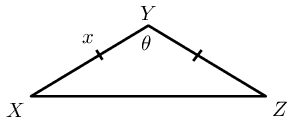If $$XY=x$$ and $$X\hat{Y}Z=\theta$$, show that $$XZ = x\sqrt{2(1- \cos \theta)}$$.

\begin{align*} XZ^2 &= 2x^2 - 2x^2 \cos \theta \\ &= 2x^2 \left(1 - \cos \theta \right) \\ \therefore XZ &= \sqrt{2x^2 \left(1 - \cos \theta \right)} \\ &= x\sqrt{2 \left(1 - \cos \theta \right)} \end{align*}

Calculate $$XZ$$ (to the nearest kilometre) if $$x=\text{240}$$ km and $$\theta = \text{132}\text{°}$$.

\begin{align*} XZ^2 &= x\sqrt{2 \left(1 - \cos \theta \right)} \\ &= 240\sqrt{2 \left(1 - \cos \text{132}\text{°} \right)} \\ &= \text{438,5}\text{ km} \end{align*}

Find the area of $$WXYZ$$ (to two decimal places):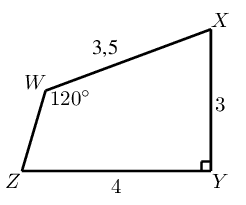\begin{align*} \frac{\sin W\hat{Z}X }{\text{3,5}} &= \frac{\sin \text{120}\text{°} }{\text{5}} \\ \therefore W\hat{Z}X &= \text{37,3}\text{°} \\ \therefore W\hat{X}Z &= \text{180}\text{°} - \text{120}\text{°} - \text{37,3}\text{°} \\ &= \text{22,7}\text{°} \\ \therefore \text{Area} WXYZ &= \frac{1}{2}(\text{3,5})(\text{5})\sin \text{22,7}\text{°} + \frac{1}{2}(4)(3)\\ &= \text{9,38}\text{ m$^{2}$} \end{align*}

Find the area of the shaded triangle in terms of $$x$$, $$\alpha$$, $$\beta$$, $$\theta$$ and $$\phi$$:\begin{align*} \text{In } \triangle ABE, \quad \frac{x}{EB} &= \cos \theta \\ \therefore EB &= \frac{x}{\cos \theta} \\ \text{In } \triangle BDC, \quad \frac{DB}{\sin \alpha} &= \frac{x}{\sin \beta} \\ \therefore DB &= \frac{x \sin \alpha }{\sin \beta} \\ \therefore \text{Area} \triangle BED &= \frac{1}{2}(BE)(BD)\sin (\beta - \theta)\\ &= \frac{1}{2} \times \frac{x}{\cos \theta} \times \frac{x \sin \alpha }{\sin \beta} \times \sin (\beta - \theta)\\ &= \frac{x^2 \sin \alpha \sin (\beta - \theta) }{2 \cos \theta \sin \beta} \end{align*}